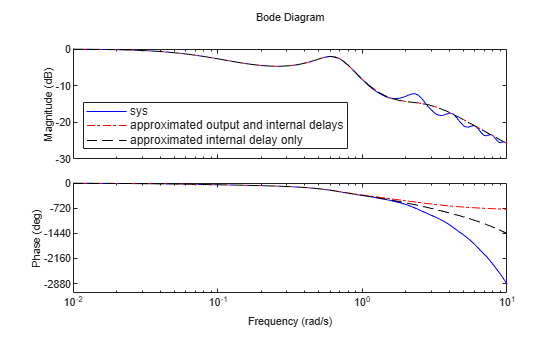# Approximate Different Delays with Different Approximation Orders

This example shows how to specify different Padé approximation orders to approximate internal and output delays in a continuous-time open-loop system.

Load a sample continuous-time open-loop system that contains internal and output time delays.

```load('PadeApproximation1.mat','sys') sys```
```sys = A = x1 x2 x1 -1.5 -0.1 x2 1 0 B = u1 x1 1 x2 0 C = x1 x2 y1 0.5 0.1 D = u1 y1 0 (values computed with all internal delays set to zero) Output delays (seconds): 1.5 Internal delays (seconds): 3.4 Continuous-time state-space model. ```

`sys` is a second-order continuous-time `ss` model with internal delay 3.4 s and output delay 1.5 s.

Use the `pade` function to compute a third-order approximation of the internal delay and a first-order approximation of the output delay.

```P13 = pade(sys,inf,1,3); size(P13)```
```State-space model with 1 outputs, 1 inputs, and 6 states. ```

The three input arguments following `sys` specify the approximation orders of any input, output, and internal delays of `sys`, respectively. `inf` specifies that a delay is not to be approximated. The approximation orders for the output and internal delays are one and three respectively.

Approximating the time delays with `pade` absorbs delays into the dynamics, adding as many states to the model as orders in the approximation. Thus, `P13` is a sixth-order model with no delays.

For comparison, approximate only the internal delay of `sys`, leaving the output delay intact.

```P3 = pade(sys,inf,inf,3); size(P3)```
```State-space model with 1 outputs, 1 inputs, and 5 states. ```
`P3.OutputDelay`
```ans = 1.5000 ```
`P3.InternalDelay`
```ans = 0x1 empty double column vector ```

`P3` retains the output delay, but the internal delay is approximated and absorbed into the state-space matrices, resulting in a fifth-order model without internal delays.

Compare the frequency response of the exact and approximated systems `sys`, `P13`, `P3`.

```h = bodeoptions; h.PhaseMatching = 'on'; bode(sys,'b-',P13,'r-.',P3,'k--',h,{.01,10}); legend('sys','approximated output and internal delays','approximated internal delay only',... 'location','SouthWest')```Notice that approximating the internal delay loses the gain ripple displayed in the exact system.